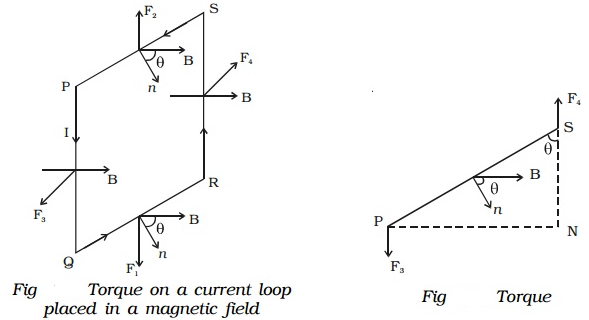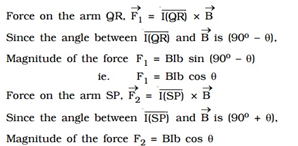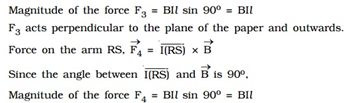Let us consider a rectangular loop PQRS of length l and breadth b (Fig). It carries a current of I along PQRS. The loop is placed in a uniform magnetic field of induction B. Let θ be the angle between the normal to the plane of the loop and the direction of the magnetic field.The force F1 and F2 are equal in magnitude, opposite in direction and have the same line of action. Hence their resultant effect on the loop is equal.Magnitude of the force F4 = BIl sin 90o = BIl

F4 acts perpendicular to the plane of the paper and inwards. The forces F3 and F4 are equal in magnitude, opposite in direction and have different lines of action. So, they constitute a couple.

Hence, Torque = BIl × PN = BIl × PS × sin θ

= BIl × b sin θ = BIA sin θ

If the coil contains n turns, τ = nBIA sin θ

So, the torque is maximum when the coil is parallel to the magnetic field and zero when the coil is perpendicular to the magnetic field.